### IMO Shortlist 2006 problem C5

Kvaliteta:
Avg: 0,0
Težina:
Avg: 8,0
An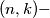$(n, k) -$ tournament is a contest with$n$ players held in$k$ rounds such that: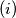$(i)$ Each player plays in each round, and every two players meet at most once.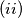$(ii)$ If player$A$ meets player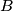$B$ in round$i$, player$C$ meets player$D$ in round$i$, and player$A$ meets player$C$ in round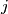$j$, then player$B$ meets player$D$ in round$j$.

Determine all pairs$(n, k)$ for which there exists an$(n, k) -$ tournament.
Izvor: Međunarodna matematička olimpijada, shortlist 2006